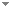Switch to: References

# Degrees bounding principles and universal instances in reverse mathematics

Annals of Pure and Applied Logic 166 (11):1165-1185 (2015)

 Open questions about Ramsey-type statements in reverse mathematics.Ludovic Patey - 2016 - Bulletin of Symbolic Logic 22 (2):151-169.Ramsey’s theorem states that for any coloring of then-element subsets of ℕ with finitely many colors, there is an infinite setHsuch that alln-element subsets ofHhave the same color. The strength of consequences of Ramsey’s theorem has been extensively studied in reverse mathematics and under various reducibilities, namely, computable reducibility and uniform reducibility. Our understanding of the combinatorics of Ramsey’s theorem and its consequences has been greatly improved over the past decades. In this paper, we state some questions which naturally arose (...) Direct download (2 more)     Export citationBookmark4 citations Dominating the Erdős–Moser theorem in reverse mathematics.Ludovic Patey - 2017 - Annals of Pure and Applied Logic 168 (6):1172-1209. Direct download (5 more)     Export citationBookmarkThe reverse mathematics of the thin set and erdős–moser theorems.Lu Liu & Ludovic Patey - 2022 - Journal of Symbolic Logic 87 (1):313-346.The thin set theorem for n-tuples and k colors states that every k-coloring of $[\mathbb {N}]^n$ admits an infinite set of integers H such that $[H]^n$ avoids at least one color. In this paper, we study the combinatorial weakness of the thin set theorem in reverse mathematics by proving neither $\operatorname {\mathrm {\sf {TS}}}^n_k$, nor the free set theorem imply the Erdős–Moser theorem whenever k is sufficiently large. Given a problem $\mathsf {P}$, a computable instance of $\mathsf {P}$ is universal (...) Direct download (2 more)     Export citationBookmark